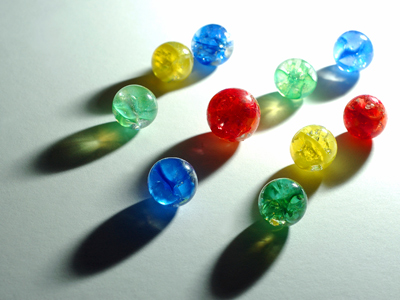If I have 10 marbles and lose 3 of them how many will I have left? It helps to work out the answer if we can see the marbles.

# Year 1 Numbers - Picture Problems

This quiz addresses the requirements of the National Curriculum KS1 Maths and Numeracy for children aged 5 and 6 in year 1. Specifically this quiz is aimed at the section dealing with solving problems using objects and pictorial representations.

Picture problems are number questions which use representative illustrations to help solve them. These representations can be useful when counting on or back, as it’s often easier to work out the answer to a problem if you can see a picture of what you’re counting!

Question 1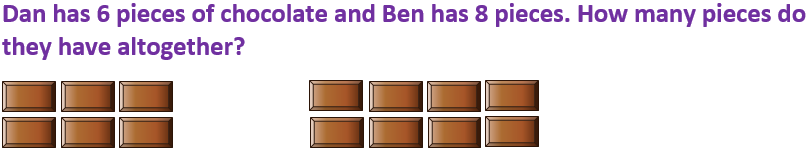14
15
16
12
6 plus 8 is 14
Question 2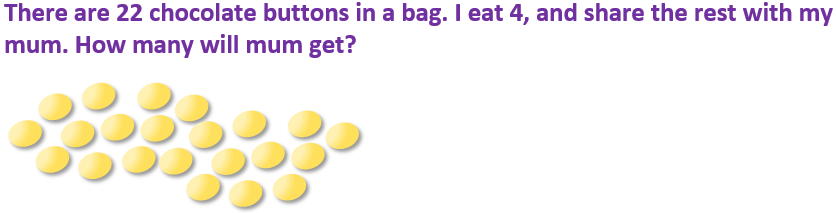11
8
10
9
22 – 4 = 18. Half of 18 is 9
Question 3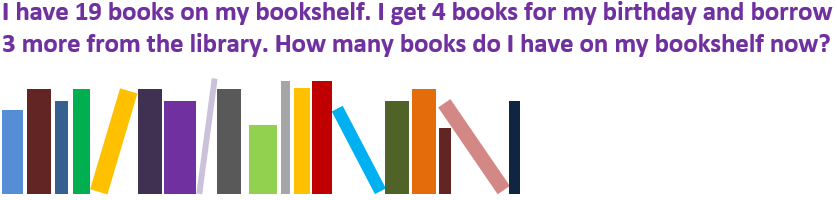25
28
24
26
19 plus 4 plus 3 is 26
Question 4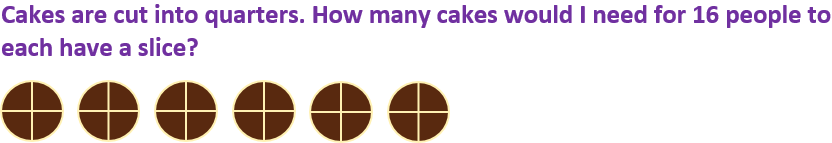4
6
5
10
There are 4 groups of 4 in 16
Question 5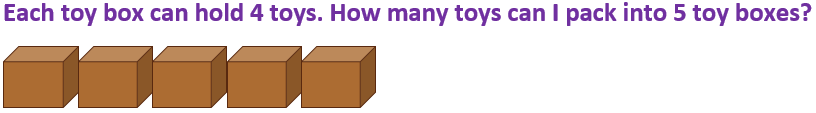16
14
22
20
5 groups of 4 make 20
Question 618
15
17
14
4 more than 10 is 14
Question 7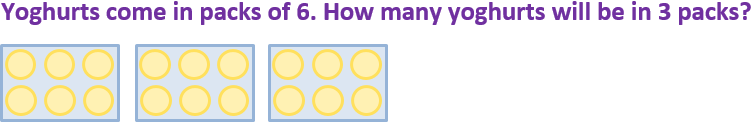20
16
18
14
3 groups of 6, or 6 + 6 + 6, is 18
Question 8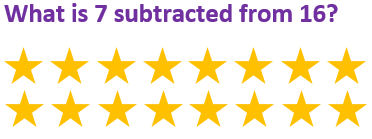8
9
7
17
If we take 7 away from the 16 stars, 9 are left
Question 9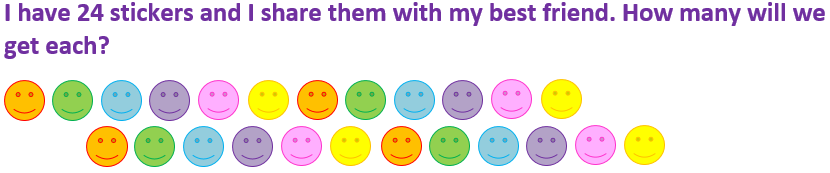14
10
6
12
Half of 24 is 12
Question 10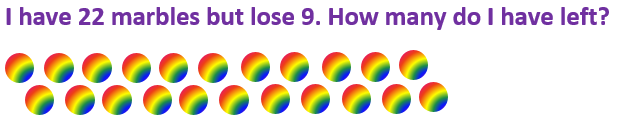3
13
26
31
9 subtracted from 22 is 13
Author:  Angela Smith